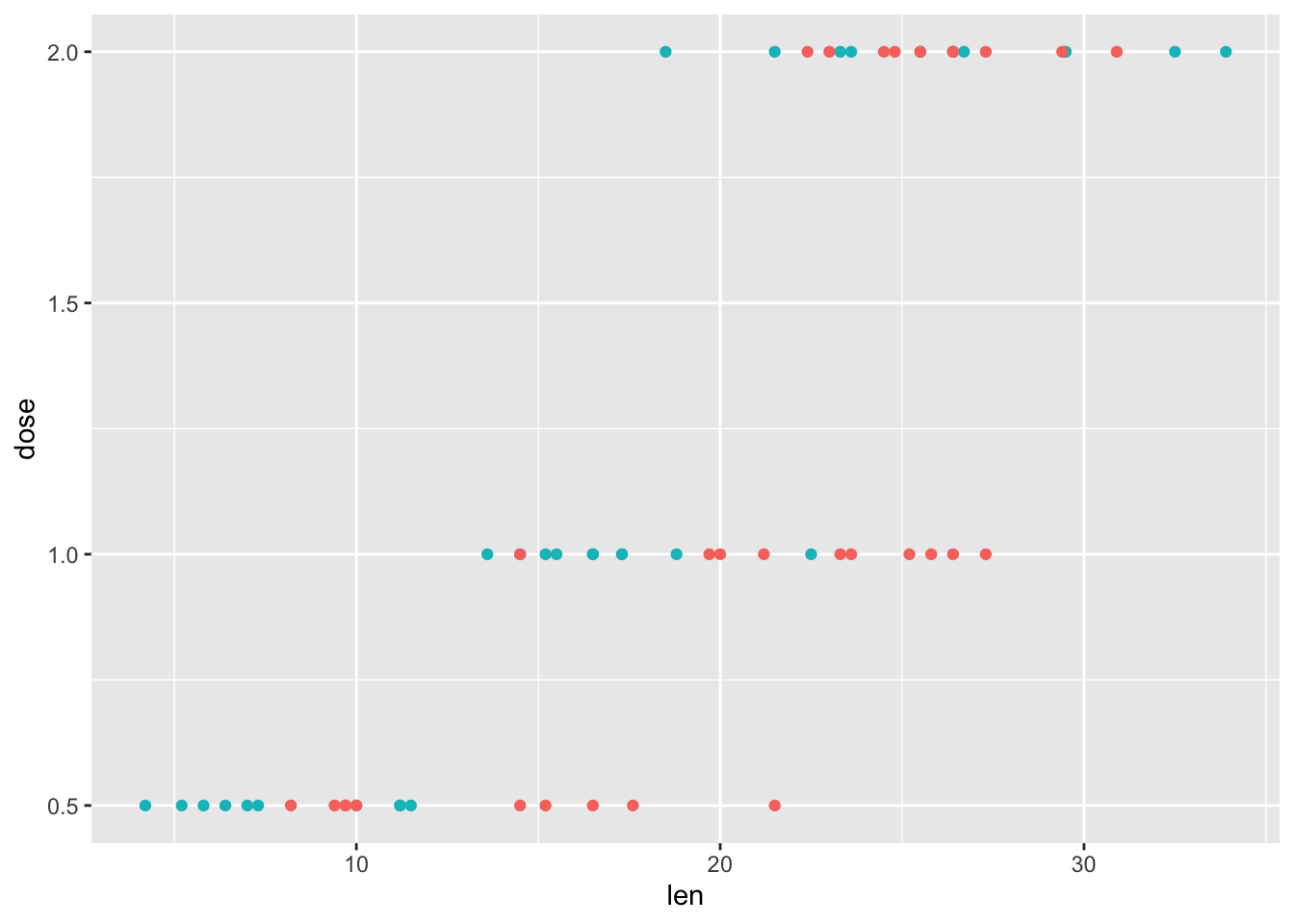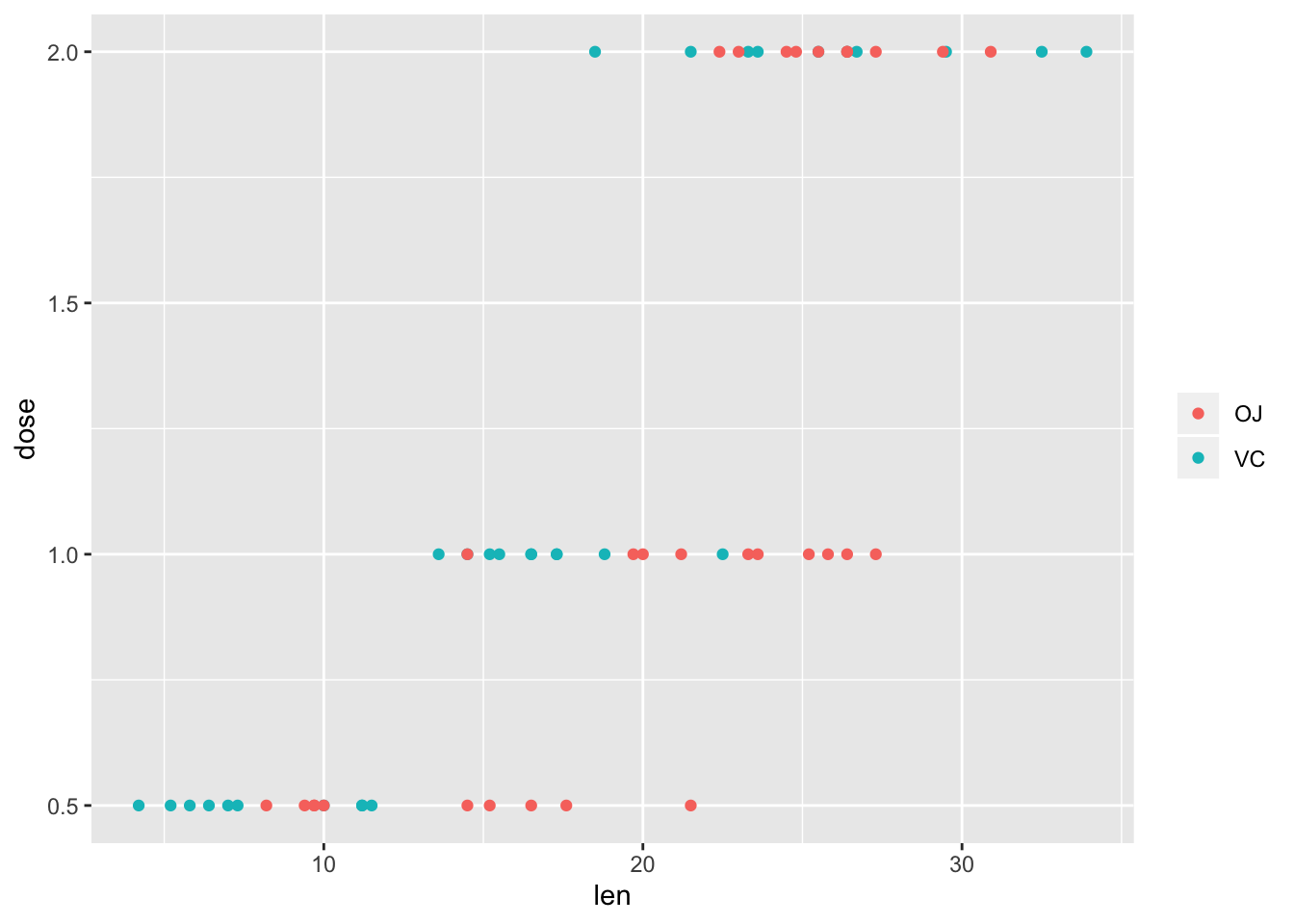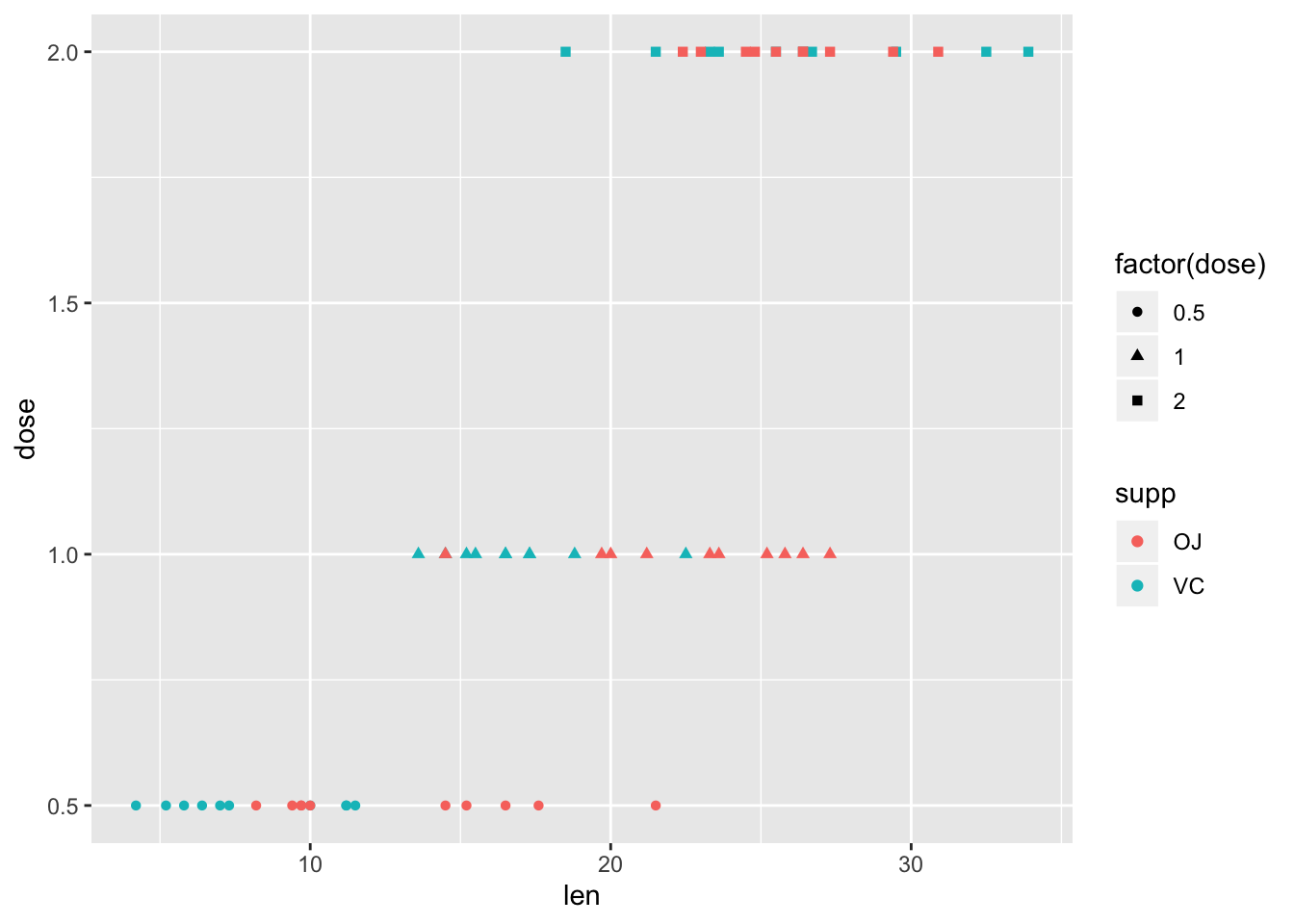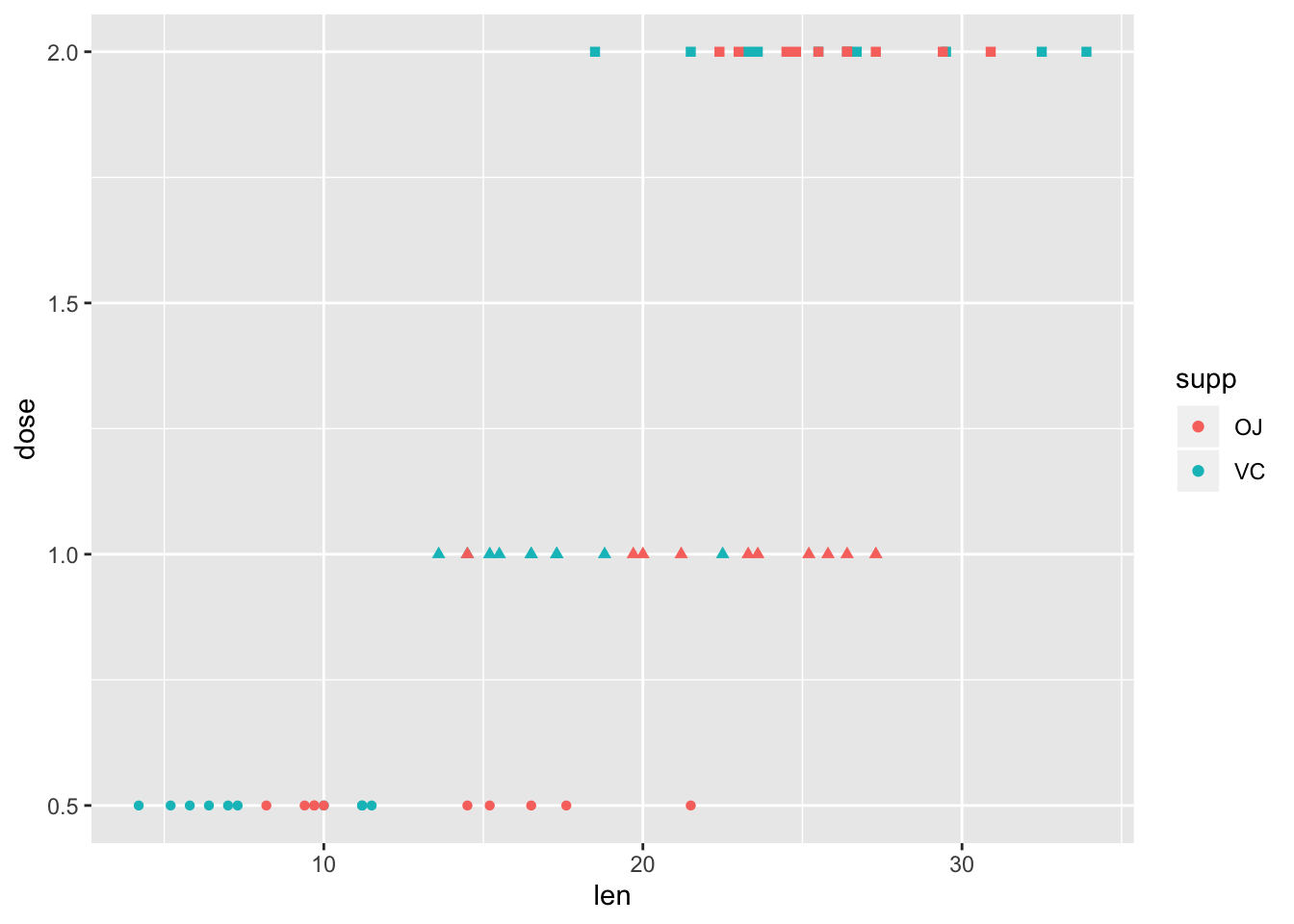## Motivation

There are some things that I always forget how to do in R. I have decided to add some of those code snippets to this blog so I that I can look them up when I need them.

## Droping variables using a vector of names

I often have a pre-specified vector of variables names that I use frequently and sometimes I want to drop those variables. The trick is to use `one_of` around the vector with variable names. The other day I spent 10 minutes trying things like `any_of` but it should be `one_of`. Now I will never forget (or if I forget then I can read my own blog)

``````vars_to_drop <- c('Wind', 'Temp')
airquality <- airquality %>%
select(-one_of(vars_to_drop))``````

## Messing with the ggplot legend

Every time I create a graph I end up hating the legend. Or maybe ‘hating’ is a strong word but at the very least I waste loads of time faffing about with it. The key to success seems to be change the options in the `theme` function.

### Removing the legend completly

In this example I probably shouldn’t delete the legend because it is useful but I wanted to use the `ToothGrowth` data becasue of its epic name.

``````ggplot(ToothGrowth, aes(x=len, y=dose, colour=supp)) + geom_point() +
theme(legend.position = 'none')``````### Removing legend title

Just removing the legend title is probably a more sensible approach.

``````ggplot(ToothGrowth, aes(x=len, y=dose, colour=supp)) + geom_point() +
theme(legend.title = element_blank())``````### Removing bits of the legend

Let’s pretend that for some stupid reason I do not only want the colour to vary by `supp` (whatever that variable is) but also the shape by the dose. I then get two legends with separate legends.

``ggplot(ToothGrowth, aes(x=len, y=dose, colour=supp, shape=factor(dose))) + geom_point() ``But maybe I don’t want all those legends!?!?!?!?! The solution was not using `theme()` anymore but the individual `scale()` functions.

``ggplot(ToothGrowth, aes(x=len, y=dose, colour=supp, shape=factor(dose))) + geom_point() + scale_shape(guide=FALSE)``### Packaged used

``library(ggplot2)``